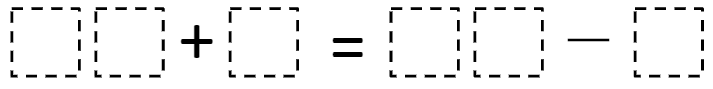Directions: Use the digits 1-9, at most once, to complete the equation.
What is the greatest result you can make?
What is the least result you can make?### Hint

What numbers work (or won’t work) for the tens digits?
Could the result have a zero in the one’s place?
Could the least result be less than 10?
Could the greatest result be greater than 100?

There are many answers. Here are some:

27 – 8 = 14 + 5

25 – 8 = 13 + 4 (or 14 + 3) ~ least result of 17 = 17

95 – 2 = 87 + 6 (or 86 + 7) ~ greatest result of 93 = 93

Source: Molly Rawding

## Order Numbers

Directions: Using the digits 0 through 9 at most one time each, place a digit …

1.2.•